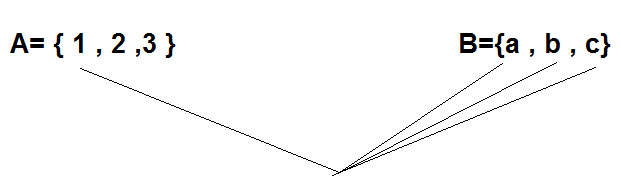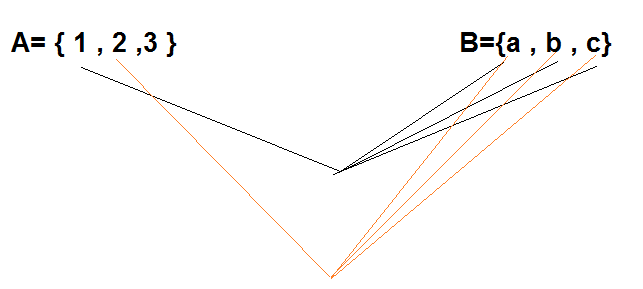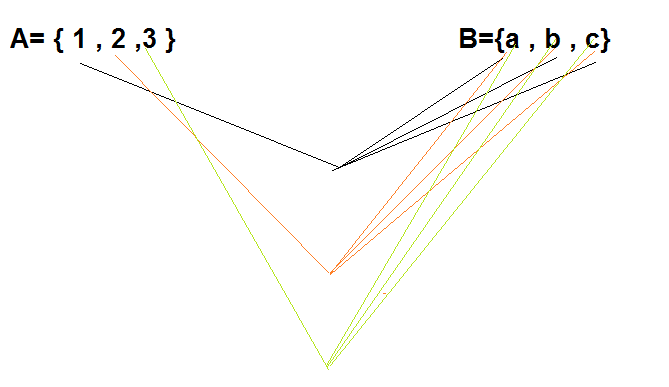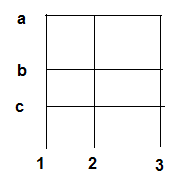# Cartesian Products of sets| Ordered Pairs

## 1. What is Cartesian Product of Sets?

Given two non-empty sets $A$ and $B$. The Cartesian product $A \times B$ is the set of all ordered pairs of elements from $A$ and $B$, i.e.,
$A \times B = \left\{ {\left( {a,b} \right):a \in A,b \in B} \right\}$
If either $A$ or $B$ is the null set, then $A \times B$ will also be empty set, i.e., $A \times B =\phi$
What is Ordered Pair in sets
An ordered pair of elements taken from any two sets P and Q is a pair of elements written in small brackets and grouped together in a particular order, i.e., (p,q), $p \in P$ and $q \in Q$ .

## Important Points on Cartesian Product of Sets

### ALgebra of ordered pairs

Two ordered pairs are equal, if and only if the corresponding first elements are equal and the second elements are also equal.
Example
Ordered pair (3,2) is equal to ordered pair (3,2)
Ordered pair (2,3) is not equal to ordered pair (3,2)
Ordered pair (1,1) is equal to ordered pair (1,1)

### Cardinality of Cartesian Product of Sets

1. If there are $p$ elements in $A$ and $q$ elements in $B$, then there will be $pq$ elements in $A \times B$, i.e., if $n\left( A \right) = p$ and $n\left( B \right) = q$, then $n\left( {A \times B} \right) = pq$.
2. If there are $p$ elements in $A$ and $q$ elements in $B$ and $r$ in $C$, then there will be $pqr$ elements in $A \times B \times C$, i.e., if $n\left( A \right) = p$ and $n\left( B \right) = q$, then $n\left( {A \times B} \right) = pq$.
3. If $A$ and $B$ are non-empty sets and either $A$ or $B$ is an infinite set , then so is $A \times B$.

### Cartesian Product with Empty sets

If either $A$ or $B$ is the null set, then $A \times B$ will also be empty set, i.e., $A \times B =\phi$
Example
A={1,2} , B={} , then $A \times B =\phi$

### Cartesian Product of a set with itself

$A \times A = \left\{ {\left( {a,b} \right):a,b \in A} \right\}$.
Example
A={1,2} then $A \times A={(1,1),(1,2),(2,1),(2,2)}$

### Cartesian product of three sets

1. $A \times A \times A = \left\{ {\left( {a,b,c} \right):a,b,c \in A} \right\}$. Here ${\left( {a,b,c} \right)}$ is called an ordered triplet
2. In general if $A_1,A_2,A_3....A_n$ are any n sets,then Cartesian product of $A_1,A_2,A_3....A_n$ and is denoted by $A_1 \times A_2 \times A_3 ....\times A_n$ and it is defined as
$A_1 \times A_2 \times A_3 ....\times A_n =\left\{(a_1 ,a_2 ,...,a_n) : a_i \in A_i , 1 \leq i\leq n \right\}$ and $(a_1 ,a_2 ,...,a_n)$ is called the ordered n-tuple

## How to find the elements of the Cartesian Product of Sets

1. Suppose we need to find the Cartesian Product of the sets A={1,2,3} and B={a,b,c}
2. Take first element of First set and then form all the ordered pair with the all the element of the second set one by one3. Ordered pair obtained from this are { (1,a),(1,b),(1,c)}
4. Now take the second element of first set and then form all the ordered pair with the all the element of the second set one by one5. Ordered pair obtained from this are { (2,a),(2,b),(2,c)}
6. Now take the third element(last element) of first set and then form all the ordered pair with the all the element of the second set one by one7. Ordered pair obtained from this are { (3,a),(3,b),(3,c)}
8. So, total ordered pair obtained are {(1,a),(1,b),(1,c),(2,a),(2,b),(2,c),(3,a),(3,b),(3,c)} and This is cartesian product of $A \times B$
9. we can also represent this cartesian product as below## Solved Examples for cartesian product

Question 1 If $P = \left\{ {1,2} \right\}$ and $Q = \left\{ {5,4,2} \right\}$, find $P \times Q$ and $Q \times P$.
Solution
$P = \left\{ {1,2} \right\}$ and $Q = \left\{ {5,4,2} \right\}$
We know that the Cartesian product $P \times Q$ of two non-empty sets $P$ and $Q$ is defined as
$P \times Q = \left\{ {\left( {p,q} \right):p \in P,q \in Q} \right\}$
Therefore,
$P \times Q = \left\{ {\left( {1,5} \right),\left( {1,4} \right),\left( {1,2} \right),\left( {2,5} \right),\left( {2,4} \right),\left( {2,2} \right)} \right\}$
$Q \times P = \left\{ {\left( {5,1} \right),\left( {5,2} \right),\left( {4,1} \right),\left( {4,2} \right),\left( {2,1} \right),\left( {2,2} \right)} \right\}$

Question 2 If $A = \left\{ { - 1,1} \right\}$, find $A \times A \times A$
Solution
It is known that for any non-empty set $A$, $A \times A \times A$ is defined as
$A \times A \times A = \left\{ {\left( {a,b,c} \right):a,b,c \in A} \right\}$
It is given that $A = \left\{ { - 1,1} \right\}$
Therefore,
$A \times A \times A\ =\left\{ \left( { - 1, - 1, - 1} \right),\left( { - 1, - 1,1} \right), \left( { - 1,1, - 1} \right),\left( { - 1,1,1} \right) ,\left( {1, - 1, - 1} \right),\left( {1, - 1,1} \right),\left( {1,1, - 1} \right) \right\}$

Question 3 If (p + 11, q +5) = (2,1), find the values of p and q.
Solution
Since the ordered pairs are equal, the corresponding elements are equal.
So, p + 11 = 2 and q +5 = 1.
Solving we get p = -9 and q = -4.

Question 4
If R is the set of all real numbers, what do the cartesian products $R \times R$ and $R \times R \times R$ represent?
Solution
The Cartesian product $R \times R$ represents the set
$R \times R={(x, y) : x, y \in R}$
which represents the coordinates of all the points in two dimensional space and the
The Cartesian product $R \times R \times R$ represents the set set
$R \times R \times R ={(x, y, z) : x, y, z \in R}$
which represents the coordinates of all the points in three-dimensional space.

## Important properties of Cartesian products of sets

i. $A \times (B \cup C) = (A \times B) \cup (A \times C)$
ii.$A \times (B \cap C) = (A \times B) \cap (A \times C)$
iii. $A \times (B - C) = (A \times B) - (A \times C)$
iv. $(A \times B) \cap ( C \times D) =(A \cap C) \times (B \cap D)$
v. if $A \subset B$ then $A \times C \subset B \times C$
vi. $A \times B \ne B \times A$
vii. $(A \times B) \times C \ne A \times (B \times C)$

### Cartesian products of sets Quiz Time

Question 1.
Let P = {2,3} and Q = {3,4}. then
A. $P \times Q = {(2,2),(2,4),(3,3), (4,4)}$
B. $P \times Q = {(2,1),(3,1), (4,2)}$$C.$P \times Q = {(2,2),(2,3),(3,4), (4,4)}$D.$P \times Q = {(2,3),(2,4),(3,3), (3,4)}$Question 2. Let A = {1, 2, 3,4} and B = {5, 7}. Then number of elements in the cartesian product$A \times B$? A. 64 B. 256 C. 8 D. 32 Question 3. which of the following is incorrect A.$A \times B= B \times A$B.$ A \times (B \cup C) = (A \times B) \cup (A \times C)$C.$ A \times (B \cap C) = (A \times B) \cap (A \times C)$D. None of the above Question 4. If A={} and B={1,2},then$n(A \times B)$A. 2 B. 0 C. 4 D. 1 Question 5. if$( \frac {a}{2} +\frac {1}{2}, b + \frac {1}{2})=(\frac {1}{4},\frac {3}{2})$A. (-1,1]) B. (0,1) C. (1,-1) D. (-1,0) Question 6. Let A = {2,3} then$A\times A\$
A. {(2,2}(3,3)}
B. {(2,2}(3,3),(2,3),(3,2)}
C. {(2,3),(3,2)}
D. None of the above